Download Presentation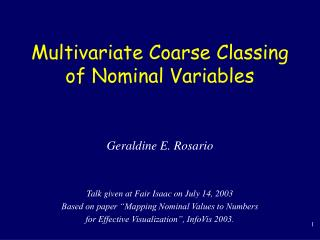Multivariate Coarse Classing of Nominal Variables

# Multivariate Coarse Classing of Nominal Variables - PowerPoint PPT Presentation

Multivariate Coarse Classing of Nominal Variables . Geraldine E. Rosario Talk given at Fair Isaac on July 14, 2003 Based on paper “Mapping Nominal Values to Numbers for Effective Visualization”, InfoVis 2003. Outline. Motivation Overview of Distance-Quantification-Classing approachI am the owner, or an agent authorized to act on behalf of the owner, of the copyrighted work described.
Download Presentation## Multivariate Coarse Classing of Nominal Variables

An Image/Link below is provided (as is) to download presentation

Download Policy: Content on the Website is provided to you AS IS for your information and personal use and may not be sold / licensed / shared on other websites without getting consent from its author.While downloading, if for some reason you are not able to download a presentation, the publisher may have deleted the file from their server.

- - - - - - - - - - - - - - - - - - - - - - - - - - E N D - - - - - - - - - - - - - - - - - - - - - - - - - -
Presentation Transcript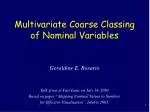### Multivariate Coarse Classing of Nominal Variables

Geraldine E. Rosario

Talk given at Fair Isaac on July 14, 2003

Based on paper “Mapping Nominal Values to Numbers

for Effective Visualization”, InfoVis 2003.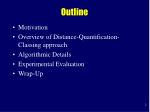Outline
• Motivation
• Overview of Distance-Quantification-Classing approach
• Algorithmic Details
• Experimental Evaluation
• Wrap-Up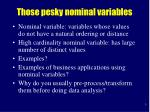Those pesky nominal variables
• Nominal variable: variables whose values do not have a natural ordering or distance
• High cardinality nominal variable: has large number of distinct values
• Examples?
• Examples of business applications using nominal variables?
• Why do you usually pre-process/transform them before doing data analysis?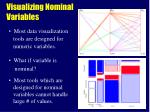Visualizing Nominal Variables
• Most data visualization
• tools are designed for
• numeric variables.
• What if variable is
• nominal?
• Most tools which are
• designed for nominal
• variables cannot handle
• large # of values.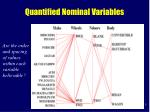Quantified Nominal Variables

Are the order

and spacing

of values

within each

variable

believable?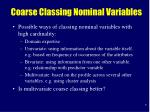Coarse Classing Nominal Variables
• Possible ways of classing nominal variables with high cardinality:
• Domain expertise
• Univariate: using information about the variable itself. e.g. based on frequency of occurrence of the attributes
• Bivariate: using information from one other variable. e.g. relationship with predictor variable
• Multivariate: based on the profile across several other variables. e.g. using cluster analysis
• Is multivariate coarse classing better?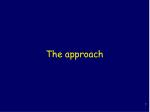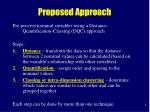Proposed Approach

Pre-process nominal variables using a Distance-Quantification-Classing (DQC) approach

Steps:

• Distance – transform the data so that the distance between 2 nominal values can be calculated (based on the variable’s relationship with other variables)
• Quantification– assign order and spacing to the nominal values
• Classing or intra-dimension clustering – determine which values are similar to each other and can be grouped together

Each step can be done by more than one technique.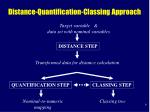Target variable &

data set with nominal variables

DISTANCE STEP

Transformed data for distance calculation

QUANTIFICATION STEP

CLASSING STEP

Nominal-to-numeric

mapping

Classing tree

Distance-Quantification-Classing Approach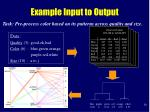Observed Counts

COLOR by QUALITY

Good Ok Bad Total

Blue 187 727 546 1460

Green 267 538 356 1161

Orange 276 411 191 878

Purple 155 436 361 952

Red 283 307 357 947

White 459 366 327 1152

Total 1627 2785 2138 6550

blue purple green red orange white

-0.02 0 -0.54 -0.5 0.55 0.57

Example Input to Output

Task: Pre-process color based on its patterns across quality and size.

Data:

Color (6) : blue,green,orange,

purple,red,white

Size (10) : a to jOther Potential Uses of DQC as Pre-Processor
• For techniques that require numeric inputs: linear regression, some clustering algorithms (can speed up calculations but with some loss of accuracy)
• For techniques that require low cardinality nominal variables: scorecards, neural networks, association rules
• FICO-specific:
• Multivariate coarse classing
• ClusterBots – nominal variables could be quantified and distance calculations would be simpler. Could be applied to mixed variables?
• Product groups, merchant groups
• Can you think of other uses?Distance Step: Correspondence Analysis
• Used for analyzing n-way tables containing some measure of association between rows and columns
• Simple Correspondence Analysis (SCA) – for 2 variables
• Multiple Correspondence Analysis (MCA) – for > 2 variables. Uses SCA.
• Focused Correspondence Analysis (FCA) – proposed alternative to MCA when memory is limited. Uses SCA.
• Reinvented as Dual Scaling, Reciprocal Averaging, Homogeneity Analysis, etc.
• Similar to PCA but for nominal variablesObserved Counts

COLOR by QUALITY

Good Ok Bad Total

Blue 187 727 546 1460

Green 267 538 356 1161

Orange 276 411 191 878

Purple 155 436 361 952

Red 283 307 357 947

White 459 366 327 1152

Total 1627 2785 2138 6550

Row Percentages

Blue 13 50 37 100

Green 23 46 31 100

Orange 31 47 22 100

Purple 16 46 38 100

Red 30 32 38 100

White 40 32 28 100

Simple Correspondence Analysis – The Basic Idea

Calculate c2 statistic (measures the

strength of association between

COLOR and QUALITY based on

assumption of independence).

Any deviation from independence

will increase the c2 value.

Can we find similar COLORs based

on its association with QUALITY?

Similar profilesSimple Correspondence Analysis – Steps

Row

percentage

matrix

Column

percentage

matrix

Normalize counts table

Similar row profiles:

(blue,purple), …

Similar column profiles:

Eigenvalues

Identify a few independent dimensions

which can reconstruct the c2 value.

(SVD, EigenAnalysis).

Coordinates for

Independent Dimensions

Dim1 Dim2

Blue - 0.02 - 0.28

Green - 0.54 0.14

Orange 0.55 0.10

Purple 0 - 0.25

Red - 0.50 0.20

White 0.57 0.19

Scale the new dimensions such that

c2 distances between row points

is maximized.Simple Correspondence Analysis – The Output
• Coordinates Matrix
• Set of independent dimensions
• Dimensions ordered by diminishing importance
• Total # of independent dimensions = min(r,c)-1
• Similar to principal components from PCA
• Eigenvalues
• Indicates the importance of each independent dimensionDistance Step Alternative: Multiple Correspondence Analysis
• Steps:
• BurtTable(rawdataMatrix)  burtMatrix
• SCA(burtMatrix)  coordMatrix, evaluesVector
• ReduceNDim(coordMatrix, evaluesVector)  coordMatrixSubset
• Input to SCA - Burt Table: crosses all variables by all variables

X1

X2

X3

X1 by X1

counts table

X1 by X2

counts table

X1

X2

X3Multiple Correspondence Analysis
• Features:
• For a given variable, determines which values are similar to each other by comparing value profiles across all other variables
• multivariate
• maximizes usage of information
• memory-intensive
• Simultaneously analyzes of all variables
• efficient calculationsReduce Number of Dimensions to Keep
• Reduce the number of independent dimensions to keep for subsequent analysis (due to large # of analysis variables and high cardinality)

eigenvalue

1 2 3 4 5

dimension #Distance Step Alternative:Focused Correspondence Analysis
• Proposed alternative to MCA when memory space is limited
• Core idea: instead of comparing value profiles across all other nominal variables, just compare value profiles across the nominal variables which are most correlated with the target variable
• Input to Simple CA:

X3

X1

X9

target

variable Xi

Xi by X3

counts table

Xi by X1

counts tableFocused Correspondence Analysis
• Steps:
• PairwiseAssociate(rawdataMatrix)  assocMatrix
• Set k (# analysis variables to use)
• FCATable(rawdataMatrix, k, assocMatrix)  fcaInputMatrix
• SCA(fcaInputMatrix)  coordMatrix, evaluesVector
• ReduceNDim(coordMatrix, evaluesVector)  coordMatrixSubsetU(R|C) Quality Color Size

Quality 1.0 0.0287 0.0028

Color 0.0173 1.0 0.1234

Size 0.0017 0.1267 1.0

FCA: Calculate Pairwise Association
• Used Uncertainty Coefficient U(R|C) to measure strength of nominal association
• Bounded [0,1]
• U(R|C)=1  value of row variable R can be known precisely given value of column variable C
• Example: U(R|C) association matrix• Set k >= 2 to ensure use of at least one analysis variable per target variable
• Cannot use a threshold on the association measureFocused Correspondence Analysis
• Features:
• One-at-a-time analysis
• Less/controllable memory usage
• Sub-optimal quantification compared to MCA
• Requires pre-processing step to determine top correlated variables per target variable
• longer run timeNominal Numeric

Blue -0.02

Green -0.54

Orange 0.55

Purple 0

Red -0.50

White 0.57

• Rec Q1 Q2 ... Score
• 0.5 -0.3 … 0.4
• -0.6 0.1 … -0.02
Quantification Step: Modified Optimal Scaling

Nominal-to-numeric

mapping

Coordinates for

Independent Dimensions

Dim1 Dim2

Blue - 0.02 - 0.28

Green - 0.54 0.14

Orange 0.55 0.10

Purple 0 - 0.25

Red - 0.50 0.20

White 0.57 0.19

Optimal

Scaling

Optimal Scaling goal: maximize the variance of the scores of the records, where score = average(qij)Quantification Step: Modified Optimal Scaling
• Problem with Optimal Scaling: perfect associations between variables are not recreated in the quantified versions
• Modified Optimal Scaling:
• Let p = # of eigenvalues = 1.0
• If p >= 1 then set
• Else setCoordinates for

Independent Dimensions

Dim1 Dim2 Counts

Blue - 0.02 - 0.28 1460

Green - 0.54 0.14 1161

Orange 0.55 0.10 878

Purple 0 - 0.25 952

Red - 0.50 0.20 947

White 0.57 0.19 1152

blue purple green red orange white

Classing Step: Hierarchical Cluster Analysis

Cluster Analysis

weighted by counts

[from FCA]100

Observed Counts COLOR by SIZE

U(R|C) = 0.1234

a b … j Total

Blue 0 8 … 1460

Green 0 2 … 1161

Orange 7 49 … 878

Purple 0 5 … 952

Red 0 0 … 947

White 6 70 … 1152

Total 13 134 … 6550

50

0

Info loss

blue purple green red orange white

Loss of Information due to Classing
• Determine variable V with highest association with target X.
• Create X by V counts table.
• Calculate total table measure of association (eg, U(X|V)).
• Starting from bottom of tree, for every pair of nodes merged,
• calculate cumulative information loss:Target variable &

data set with nominal variables

DISTANCE STEP

Transformed data for distance calculation

QUANTIFICATION STEP

CLASSING STEP

Nominal-to-numeric

mapping

Classing tree

Distance-Quantification-Classing ApproachExperimental Evaluation
• Wrong quantification and classing will introduce artificial patterns and cause errors in interpretation
• Evaluation measures:
• Believability
• Quality of Visual Display
• Quality of classing
• Quality of quantification
• Space – FCA less space
• Run time – MCA faster

perception

statistical

computationalBelievability and Quality of Visual Display
• Given two displays resulting from different nominal-to-numeric mappings:
• Which mapping gives a more believable ordering and spacing?
• Based on your domain knowledge, are the values that are positioned close together similar to each other?
• Are the values that are positioned far from the rest of the values really outliers?
• Which display has less clutter?Automobile Data: MCA

Are these

patterns

believable?Automobile Data: FCA

Are these

patterns

believable?PERF Data: Alphabetical

Region-Country:

1-many

Country-Product:

many-many

Are these

associations

preserved and

revealed?PERF Data: FCA

Region-Country:

1-many

Country-Product:

many-many

Are these

associations

preserved and

revealed?Quality of Classing
• Classing A is better than classing B if, given a classing tree, the rate of information loss with each merging is slower

Information loss

due to classing

for one variable 

[The lower the line,

the slower the info loss,

the better the classing.]

Calculate

difference

between

the lines. Which classing is better … depends on dataset

Distribution of

difference

between

the lines.Quality of Quantification
• A quantification is good if …
• If data points that are close together in nominal space are also close together in numeric space
• If two variables are highly associated with each other, then their quantified versions should also have high correlation.MCA gives better quantification

Average

Squared

Correlation

[higher value =

better quantification]

Correlation between

MCA and FCA scales

[how close are FCA

scales to MCA scales]Going back to Multivariate Coarse Classing
• Other issues:
• Missing values
• Mixed or numeric variables as analysis variables
• Nominal values with small counts
• Robustness of quantification and classingCan you think of other uses of DQC at FICO?
• For techniques that require numeric inputs: linear regression, some clustering algorithms (can speed up calculations but with some loss of accuracy)
• For techniques that require low cardinality nominal variables: scorecards, neural networks, association rules
• FICO-specific:
• Multivariate coarse classing
• ClusterBots – nominal variables could be quantified and distance calculations would be simpler. Could be applied to mixed variables?
• Product groups, merchant groups
• ???????Implementation
• SAS version exists
• PROC CORRESP, PROC CLUSTER, PROC FREQ
• C++ version in developmentSummary
• DQC is a general-purpose approach for pre-processing nominal variables for data analysis techniques requiring numeric variables or low cardinality nominal variables
• DQC – multivariate, data-driven, scalable, distance-preserving, association-preserving
• FCA is a viable alternative to MCA when memory space is limited
• Quality of classing and quantification
• depends on strength of associations within the data set.
• is in the eye of the userYippee, it’s over!

Original InfoVis2003 paper: Mapping Nominal Values to Numbers for Effective Visualization.

http://davis.wpi.edu/~xmdv/documents.html

XmdvTool Homepage:

http://davis.wpi.edu/~xmdv

xmdv@cs.wpi.edu

Code is free for research and education.References
• [Gre93] GREENACRE, M.J., 1993, Correspondence Analysis in Practice, London :Academic Press
• [Gre84] Greenacre, M. (1984), Theory and Applications of Correspondence Analysis, London: Academic Press
• [Sta] StatSoft Inc. Correspondence Analysis. http://www.statsoftinc.com/textbook/stcoran.html
• [Fri99] Friendly, Michael. 1999. "visualizing Categorical Cata." In Sirken, Monroe G. et. al. (eds). Cognition and Survey Research. New York: John Wiley & Sons.
• [Kei97] Keim D. A.: Visual Techniques for Exploring Databases, Invited Tutorial, Int. Conference on Knowledge Discovery in Databases (KDD'97), Newport Beach, CA, 1997.
• [Hua97b] Zhexue Huang. A Fast Clustering Algorithm to Cluster Very Large Categorical Data Sets in Data Mining (1997)
• SAS Manuals (PROC CORRESP, PROC CLUSTER, PROC FREQ)What input tables can SCA accept?
• In general, SCA can use as input any table that has the properties:
• The table must use the same physical units or measurements, and
• The values in the table must be non-negative.

The FCA input table satisfies these properties.Uncertainty Coefficient U(R|C)

Source: SAS Proc FreqRec Q1 Q2 ... Score

• 0.5 -0.3 … 0.4
• -0.6 0.1 … -0.02

Pair Sqr(Correlation)

Q1,score 0.36

Q2,score 0.49

average=___

Average Squared Correlation
• Given the raw data matrix R=[rij], where the columns represent the variables. Create new matrix Q=[qij] where qij.=quantified version of rij.. Let Qj=jth column of Q.
• For each record i, calculate scorei=average(Sj qij )
• For each variable j, calculate corrj=correlation(Qi,score)
• Calculate average of the squared correlation.

Source: [Gre93]